## How to use fixed point theorems

### Quick description

Let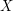be a set and let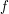be a function fromto. A fixed point ofis an element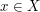such that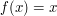. A fixed point theorem is a theorem that asserts that every function that satisfies some given property must have a fixed point. If you have an equation and want to prove that it has a solution, and if it is hard to find that solution explicitly, then consider trying to rewrite the equation in the formand applying a fixed point theorem. This method can be applied not just to numerical equations but also to equations involving vectors or functions. In particular, fixed point theorems are often used to prove the existence of solutions to differential equations.

### Prerequisites

A knowledge of some of the main fixed point theorems (though these are also discussed in the article, and links are given to Wikipedia articles about them).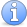Incomplete This article is incomplete. There should be several examples. In particular, there should be at least one example for each of the fixed point theorems mentioned below.

### General discussion

Some of the major fixed point theorems that can be used in analysis and topology: the Brouwer fixed point theorem, the Lefschetz fixed point theorem, the contraction mapping theorem, and the Schauder fixed point theorem.

### Example 1

Let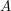be an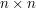matrix with non-negative entries. A result with many applications is thatmust have an eigenvector with non-negative coefficients.

To prove this, let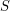be the subset of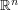that consists of all vectors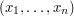such that each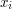is non-negative and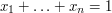. If there exists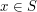such that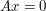, then we are done. Otherwise, we know that for every,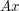has non-negative entries, not all of them zero. Let us write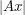for the sum of the coefficients of. Then the map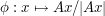is a continuous map fromto.

Now geometricallyis a simplex of dimension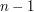, and therefore it is homeomorphic to a ball of dimension. The Brouwer fixed point theorem implies that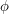has a fixed point, so there must be somesuch that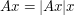. Thisis an eigenvector with eigenvalue, so the result is proved.

### Example 2

Letbe a Banach space, and let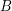andbe linear operators on. Ifis invertible and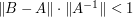, then the Contraction Mapping Theorem can be used to show thatis also invertible. Informally, this says that ifclosely approximates an invertible operator, thenis invertible.

To show thatis invertible, we will show that for each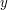in, there is a uniquesuch that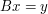. Letbe an element of. If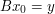for some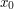in, then we have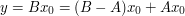. Multiplying by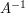, we have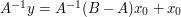. To simplify notation, write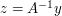and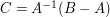, so that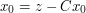.

Now we'll define a functiononby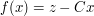. If we can show thatis a contraction mapping, then the fixed point ofwill be, asgives us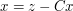.

Letandbe elements of. Then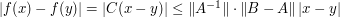. By assumption,, sois a contraction mapping, which gives us the desired fixed point.

The proof of the contraction mapping theorem proceeds by iterating the contraction. In this case, since the contraction is a linear map, one can do this explicitly and see what one obtains. The first few iterates of the functionabove are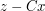,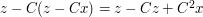,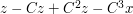, and we see that, because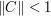, this sequence is converging to the point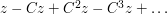.

Ifis the identity and, then this is particularly easy to see directly: the inverse of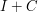is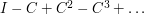, and one can justify it by noting that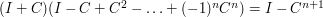, which converges to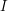. One can deduce the general case from this too, since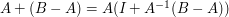. Therefore, the contraction mapping theorem is not essential in this example.Attention This article is in need of attention. It would be nice to have an example of the contraction mapping theorem applied in a less linear context so that one can't just write down a solution anyway.

### An application of the contraction mapping principle

A nice application of this principle is Picard's theorem about the existence of solutions to an initial value problem for a first order, ordinary differential equation. The map involved is highly non linear and the solution cannot be written down explicitily as in example 2. The idea is to convert the differential equation into an integral equation. The solution is then a fixed point for the operator that rules the integral equation. I just realized that there is a reference to this kind of trick at the end of the quick description above.

### An application of Schauder's fixed point theorem

An application of this result is Lomonosov's remarkable theorem about the existence of invariant subspaces for an operator on a Banach space that commutes with a non zero compact operator. It would be nice to write an article on this. Also, it could be linked from other articles like

analysis > Operator theory

How to prove the existence of > Invariant subspaces

### Fixed point theorems for set valued maps

I would like to mention that this kind of results, in particular Kakutani's fixed point theorem, can be used to produce invariant subspaces for operators on Banach spaces. Added later: where I said Kakutani, I meant to say Ky Fan.

### Universal mappings and universal morphisms

There are good reasons to study not just the fixed point property (fpp) but the common generalization of fpp and the covering dimension theory, namely the universal functions, and even the universal (and couniversal) morphisms for general categories. (Since monoids are 1-object categories, one may also focus on the universal elements of monoids).

## Post new comment

(Note: commenting is not possible on this snapshot.)

Before posting from this form, please consider whether it would be more appropriate to make an inline comment using the Turn commenting on link near the bottom of the window. (Simply click the link, move the cursor over the article, and click on the piece of text on which you want to comment.)